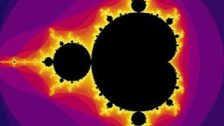# Visualising and Representing Complex Numbers### Visualising and Representing Complex Numbers

If you have never met complex numbers before, these problems offer a gentle introduction before challenging you to use different representations to visualise what happens when complex numbers are manipulated in different ways.

### A Brief Introduction to Complex Numbers

##### Age 14 to 18Challenge Level
In this problem, we define complex numbers and invite you to explore what happens when you add and multiply them.

### A Brief Introduction to the Argand Diagram

##### Age 14 to 18Challenge Level
Complex numbers can be represented graphically using an Argand diagram. This problem explains more...

### Complex Squares

##### Age 16 to 18Challenge Level
What happens when we square complex numbers? Can the square of a complex number be real?